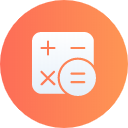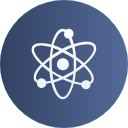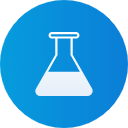No products in the cart.

# Class 11 Applied Maths Sample Papers, Important Questions, Notes and Videos

Practice resources for CBSE Class 11 Applied Maths like sample papers, mock tests, and exclusive case study questions are available on myCBSEguide.

Price 349
Valid till March 31, 2024

#### Other Useful Resourses

The Senior Secondary Applied Maths course is designed to develop mathematical language and symbolism to communicate and relate everyday experiences mathematically. This is what makes it different from pure mathematics. Practice resources for CBSE Class 11 Applied Mathematics/Maths like sample papers, mock tests, and exclusive case study questions are available on myCBSEguide.

## What is the purpose of Applied Mathematics?

Mathematics is a highly prospective subject. That is to say, it is widely used in higher studies not only in the science stream but almost in every field like Economics, Commerce, Social Sciences, and many others. Secondary School Education prepares students to explore future career options after graduating from the school. It has been observed that the syllabus of Mathematics meant for Science subjects may not be appropriate for the students pursuing Commerce or Social Science-based subjects in university education. Since Mathematics holds a special position in all the three streams of the academic curriculum, therefore the Central Board of Secondary Education has developed one more elective course in the Mathematics syllabus for Senior Secondary classes. This means from Class 11 Applied Mathematics and Mathematics will be two different elective options for the students. This is done with an aim to provide students with relevant experience in Mathematics that can be used in fields other than Physical Sciences.

### Objectives of Applied Maths (Class 11 Applied Maths)

The Senior Secondary Applied Maths course is designed to develop substantial mathematical skills and methods needed in other subject areas. It is expected that the subject is taught connecting concepts to the applications in various fields. The objectives of the course areas are as follows:

1.  To develop an understanding of basic mathematical and statistical tools and their applications in the field of commerce (business/ finance/economics) and social sciences.
2. To model real-world experiences/problems into mathematical expressions using numerical/algebraic/graphical representation.
3. To make sense of the data by organizing, representing, interpreting, analyzing, and making meaningful inferences from real-world situations.
4. To develop logical reasoning skills and apply the same in simple problem-solving.
5. To reinforce mathematical communication by formulating conjectures, validating logical arguments, and testing hypotheses.
6. To make connections between Mathematics and other disciplines.

## What is the difference between Mathematics and Applied Mathematics?

Pure Maths is mathematics in wholeness, i.e., absolutely maths that may not be feasible for its practical use because it is separate from the physical world. Pure maths involves problem-solving, finding facts, and question-answers that don’t depend on the world around us but on the rules of mathematics itself. Contrarily, applied maths is mathematics for practical use. It is that domain where we can find an application of mathematics to real-world problems. Applied maths tries to model, predict and explain things in the real world. Statistics or probability theory are an example of Applied Maths.

### How will Applied Mathematics in senior secondary help the students?

• Diversified Field: Topics covered in two years (of senior secondary) aims to enable students to use mathematical knowledge in the field of business, economic and social sciences. It aims to promote appreciation of mathematical power and simplicity for its countless applications in diverse fields.
• Mathematical Language: The CBSE senior secondary course continues to develop mathematical language and symbolism to communicate and relate everyday experiences mathematically.
• Reasoning Skills: The applied maths course reinforces the logical reasoning skills of formulating and validating mathematical arguments, framing examples, and finding counterexamples. It encourages students to engage in mathematical investigations and to build connections within mathematical topics and with other disciplines.
• Interpretation & Problem-Solving: The course prepares students to use algebraic methods as a means of representation and as a problem-solving tool. It also enables students to interpret two-dimensional geometrical figures using algebra and to further deduce properties of geometrical figures in a coordinate system.
• Inferential Learning: The course content will help students to develop a sound understanding of descriptive and inferential statistics which they can use to describe and analyze a given set of data and to further make meaningful inferences out of it.
• Data-Handling: Data-based case studies from the field of business, economics, psychology, education, biology, and census data will be used to appreciate the power of data in contemporary society.

## Topics Covered Under Class 11 Applied Maths

Chapters covered under CBSE Class 11 Applied Mathematics are as follows:

• Numbers
• Indices and Logarithms
• Numerical Applications
• Mensuration
• Sets
• Relations
• Sequences and Series
• Permutations and Combinations
• Mathematical and Logical Reasoning
• Functions
• Limits and Continuity
• Differentiation
• Probability
• Descriptive Statistics
• Compound Interest and Annuity
• Taxation
• Utility Bills
• Straight Lines
• Circles and Parabola

## Class 11 Applied Maths Resources on myCBSEguide

One of India's top-rated educational apps, myCBSEguide, is known for being the best guide for CBSE courses. We have updated modules for almost every secondary and senior secondary subject in our CBSE course field. It has separate modules for senior secondary Maths and Applied Maths which becomes a one-stop solution for the students. Our resources for class 11 Applied Maths include CBSE Syllabus, CBSE Sample Papers, Case Study Questions, Mock Tests, etc.

• #### Class 11 Applied Mathematics Case Study Questions

Finding good quality Class 11 Applied Mathematics case study questions can be quite challenging. This is because case study questions can be quite marked gaining, and hence a complete understanding of such Class 11 Applied Mathematics case study question is much needed. myCBSEguide is the only app where you’ll find …

• #### CBSE Class 11 Applied Mathematics Syllabus 2022-23

CBSE Class 11 Applied Mathematics Syllabus 2022-23 includes Numbers, Quantification and Numerical Applications, Basics of Financial Mathematics, Coordinate Geometry etc for the session 2022 – 2023. Here is the detailed syllabus. To download class 11 Applied Mathematics CBSE latest sample question papers for the 2023 exams, please install the myCBSEguide …### Student Subscription

#### More Courses349

### Physics349

### Chemistry349

### Mathematics349